exploring the undesigned
intelligence of the numberverse

lists/generators: Perfect Squares, Composites, Primes"...out all day long.
All day long,
it goes on and on"

The First 1,000,000...

Lists of Composites

Generate Your Own Up to 1027...

Perfect Square Generator

Prime Factors Generator

Prime Generator

Experimental Calculators

Secrets of the Spiral

Proximate-Prime Polynomials

A proximate-prime polynomial is simply a quadratic equation - a finite polynomial of the second degree - that is derived from four successive (proximate, or neighboring) primes. Proximate-prime polynomials are interesting because they exhibit much greater prime densities than other polynomials.

The high primality of prime-derived quadratic sequences

What is a "perfect prime polynomial"?

What is polynomial pronic alignment?

Use an Excel spreadsheet to explore polynomial primes!

Catch the Wave...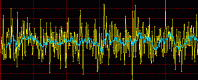When you graph primes against an X-axis that treats the expanding interval between successive perfect squares as a constant unit subdivided into equal parts, you produce a distinctive wave form for primes and prime factors.

Try out an ingenious Excel worksheet to see the wave!

Fixing Fermat’s Factorization Method

The Magic Square of Subtraction...

For every composite number that is not itself a perfect square there exists a pair of nonconsecutive perfect squares whose difference is equal to the composite. Even before we get to the subject of factorization, the consequences of this observation are fascinating and far-reaching.

Q-Paired Primes...

It began with an exploration of biquadratic paired primes: 2 primes separated by the equivalent of exactly 2 quadratic intervals.... Then the investigation took the logical next level by asking the question: Are there prime pairs that are separated by other, greater multiples of the quadratic interval? And if there are, what are the frequency characteristics by interval size and perfect square offset? The results are in, with charts, an Excel visualization, and masses of half-digested data...!

Two "new" rules of perfect squares

How I learned not to be afraid of big numbers

It's a question of magnitude...

Using Excel as a tool for number theory

Find examples throughout this site that demonstrate using VBA code with worksheets and graphing - including generating primes, perfect squares, and composites, doing modular arithmetic, calculating GCDs, and more....

 Tools for Testing Natural Numbers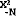MinusSquareMiller-Rabin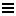FermaticTest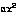QTest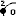RadiusTest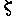ZetaTest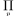PrimeTestMinusSquare: Alternative Factoring Algorithm (beta) The magic square of subtraction has given birth to a baby factoring algorithm.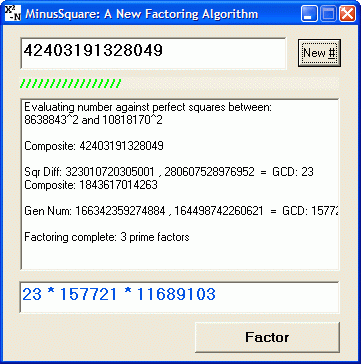Miller-Rabin Primality Test: Demo with source code (beta) Desktop program and complete project source code for implementing the gold standard in primality testing. A fast and reliable test for numbers up to 1027-1 (that's 1 with 26 9s - a prime number...!). (The project illustrates how to use a legacy language, VB6, not designed for big integers. It includes modular exponentiation code by DI Management Cryptography Software.)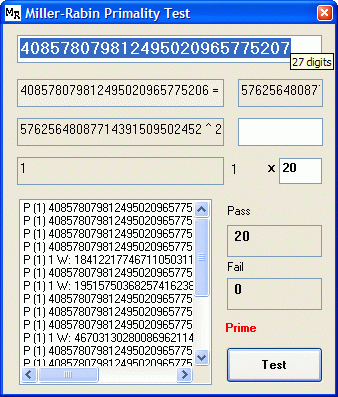FermaticTest: Prime and Pseudoprime Calculator "Fermatic" is a made-up word: Fermat + Automatic. This tool takes Fermat's great theorem to the limit, with some experiments to weed out pesky pseudoprimes. Rapidly generate prime, pseudoprime, and composite data.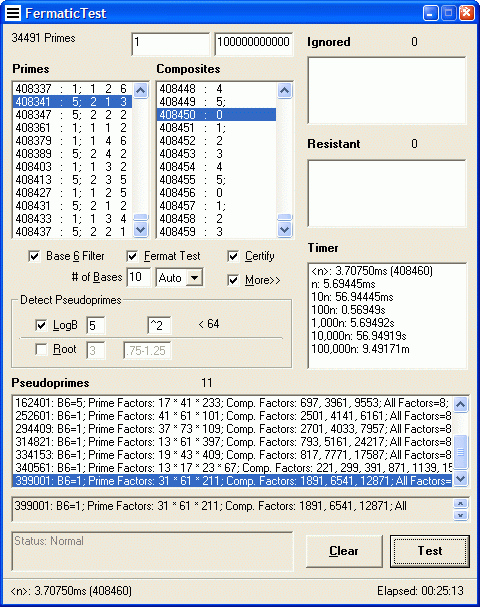Download (34KB)QTest: Quadratic Sequence Calculator Enter 3 or 4 numbers in a sequence and find out what the next 10, next 1,000, or next 10,000 values are. QTest lets you derive a quadratic equation from the values you input (and solve the equation's roots). Then you can use this polynomial to generate and analyze long number sequences for primality. (See Robert Sacks' method for quadratic derivation, used in Vortex.)RadiusTest: Polynomial Line and Curve Calculator Calculate prime and composite distribution in the Sacks Number Spiral by offset (curved series) and angle (straight series).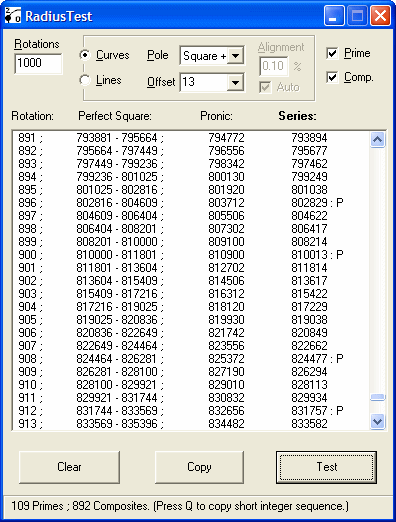Download (22KB)ZetaTest: Infinite Series Calculator Calculate the products of infinite series using almost any inputs you can think of. Generates real number zeta series, demonstrating the calculation of many important constants - including e, pi, and phi, the Basel equality and Apéry's constant.To calculate pi, use the screenshot settings Download (23KB)PrimeTest: Prime and Composite Analyzer Prime and composite number calculator (with prime and nonprime factor analyzer and prime number generator).Download (31KB) Tools that are digitally signed are published by: Wordwise SolutionsRecommended sites:

Divisor Plot

Interesting Graphing Applets